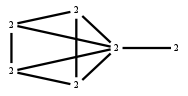The Markov Bases Database

G158g_bin

The binary graphical model of G158.

It is a hierarchical model of 6 variables. The dimension of the model is 19.The cardinality of the statespace is 64.

Properties of the Markov basis

 Markov degree 4 368

 degree # of gen. 2 4 288 80

The model has the following properties:

• All variables are binary.
• It is a graphical model.
• The semigroup is normal.FILES

 Markov basis: sufficient statistics matrix: G158g_bin.mar (69.37 kb) G158g_bin.mat (4.54 kb) G158g_bin.mod (55 b) G158g_bin.tar.gz (8.69 kb)